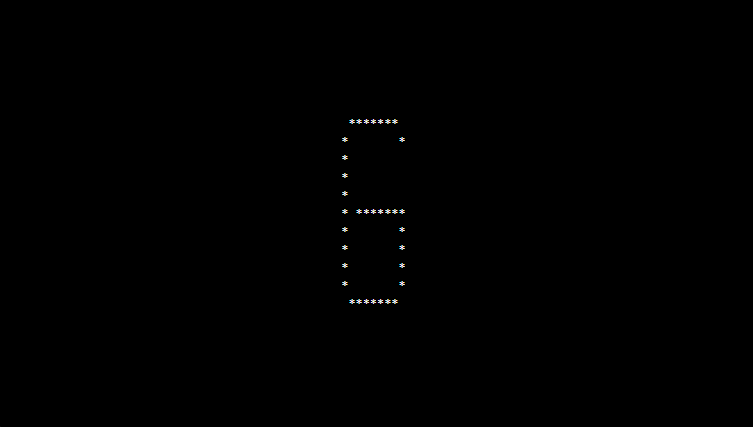# PHP program to print alphabet pattern G

In this article write a PHP program to print alphabet pattern G. This Program first takes the numbers of rows and then prints pattern using nested for loops.

## PHP program to print alphabet pattern G

```<?php
echo "<pre>";
for (\$row = 0; \$row < 11; \$row++) {
for (\$col = 0; \$col <= 11; \$col++) {
if ((\$col == 1 AND \$row != 0 AND \$row != 10) OR ((\$row == 0 OR \$row == 10) AND \$col > 1 AND \$col < 9) OR (\$row == 5 AND \$col > 2 AND \$col < 10) OR (\$col == 9 AND \$row != 0 AND \$row != 2 AND \$row != 3 AND \$row != 4 AND \$row != 10)) {
echo "*";
} else {
echo "&nbsp;";
}
}
echo "<br/>";
}
echo "</pre>";
?>
```

### Output: